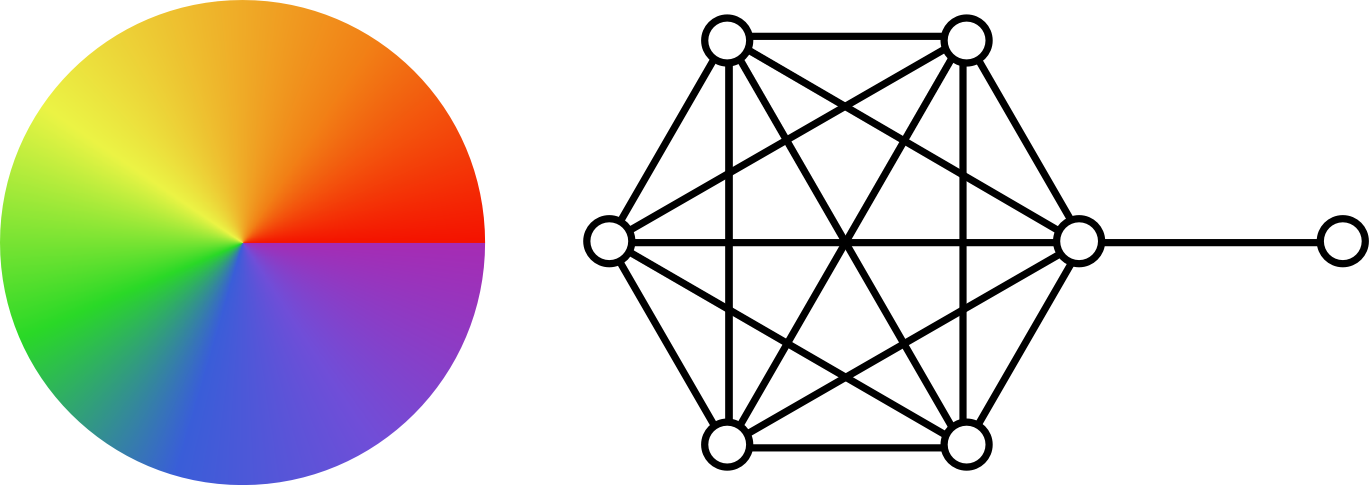# The Colorful Lollipop

Probability Level 2

What is the least number of colors needed to color each vertex of the graph below such that no two adjacent vertices have the same color?Note: In graph theory, two vertices are adjacent if they are connected by an edge.

×